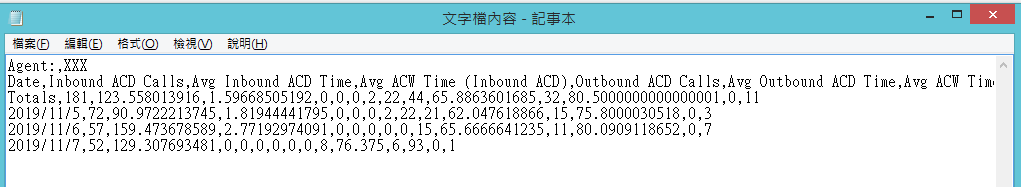1

用VBA將TXT檔匯入EXCLE

Sub InOutbound_Daily()

Dim myTxtFile As String
Dim myBuf1(4) As String
Dim myBuf2(16) As String
Dim myFName As String
Dim i As Long
Dim j As Integer
Dim k As Integer
Dim myFNo As Integer

myFNo = FreeFile
Application.ScreenUpdating = False
myFName = Dir(ActiveWorkbook.Path & "\InOutbound_Daily\" & "InOutbound_Daily*.txt")
Worksheets("InOutbound_Daily").Activate

Cells(1, 1).Value = "Agent"
Cells(1, 2).Value = "Date"
Cells(1, 3).Value = "Inbound ACD Calls"
Cells(1, 4).Value = "Avg Inbound ACD Time"
Cells(1, 5).Value = "Avg ACW Time (Inbound ACD)"
Cells(1, 6).Value = "Outbound ACD Calls"
Cells(1, 7).Value = "Avg Outbound ACD Time"
Cells(1, 8).Value = "Avg ACW Time (Outbound ACD)"
Cells(1, 9).Value = "Extn In Calls"
Cells(1, 10).Value = "Avg Extn In Time"
Cells(1, 11).Value = "Extn Out Calls"
Cells(1, 12).Value = "Avg Extn Out Time"
Cells(1, 13).Value = "External Extn Out Calls"
Cells(1, 14).Value = "Avg External Extn Out Time"
Cells(1, 15).Value = "Assists"
Cells(1, 16).Value = "Trans Out"

i = 1

Do While myFName <> ""

myTxtFile = ActiveWorkbook.Path & "\InOutbound_Daily\" & myFName

Open myTxtFile For Input As #myFNo

Input #1, myBuf1(1), myBuf1(2), myBuf1(3)
For k = 1 To 30
Input #1, myBuf1(4)
Next k

Do Until EOF(1)

Input #1, myBuf2(3), myBuf2(4), myBuf2(5), myBuf2(6), myBuf2(7), myBuf2(8), myBuf2(9), myBuf2(10), myBuf2(11), myBuf2(12), myBuf2(13), myBuf2(14), myBuf2(15), myBuf2(16)

i = i + 1
For j = 3 To 16
Cells(i, 1) = myBuf1(2)
Cells(i, 2) = myBuf1(4)
Cells(i, j) = myBuf2(j)
Next j

Loop
Close #myFNo
myFName = Dir()
Loop

End SubAgent:,XXX
Date,Inbound ACD Calls,Avg Inbound ACD Time,Avg ACW Time (Inbound ACD),Outbound ACD Calls,Avg Outbound ACD Time,Avg ACW Time (Outbound ACD),Extn In Calls,Avg Extn In Time,Extn Out Calls,Avg Extn Out Time,External Extn Out Calls,Avg External Extn Out Time,Assists,Trans Out
Totals,181,123.558013916,1.59668505192,0,0,0,2,22,44,65.8863601685,32,80.5000000000000001,0,11
2019/11/5,72,90.9722213745,1.81944441795,0,0,0,2,22,21,62.047618866,15,75.8000030518,0,3
2019/11/6,57,159.473678589,2.77192974091,0,0,0,0,0,15,65.6666641235,11,80.0909118652,0,7
2019/11/7,52,129.307693481,0,0,0,0,0,0,8,76.375,6,93,0,1slime iT邦大師 1 級 ‧ 2019-11-09 10:05:30 檢舉

1. 每一列的欄沒有對齊, 可能迴圈的列跟欄有一項寫反了, 而預期列加一造成欄也加一.
2. 有個奇怪的 k = 1 To 30 重複讀 30 次?
3. 欄數 16 , 建議讀到空欄就停止讀取的處理.
4. 有些值被換算成日期, 建議對該欄設定儲存格格式.

@浩瀚星空

1 個回答

3

Line input #把單一行文字讀進變數中，再用Split()切割。

Sub InOutbound_Daily()
Dim myTxtFile As String
Dim myFName As String
Dim i As Long
Dim myFNo As Long
Dim sAgent As String, myRec As Variant

myFNo = FreeFile
Application.ScreenUpdating = False
myFName = Dir(ActiveWorkbook.Path & "\InOutbound_Daily\" & "InOutbound_Daily*.txt")
Worksheets("InOutbound_Daily").Activate

Cells(1, 1).Value = "Agent"
Cells(1, 2).Value = "Date"
Cells(1, 3).Value = "Inbound ACD Calls"
Cells(1, 4).Value = "Avg Inbound ACD Time"
Cells(1, 5).Value = "Avg ACW Time (Inbound ACD)"
Cells(1, 6).Value = "Outbound ACD Calls"
Cells(1, 7).Value = "Avg Outbound ACD Time"
Cells(1, 8).Value = "Avg ACW Time (Outbound ACD)"
Cells(1, 9).Value = "Extn In Calls"
Cells(1, 10).Value = "Avg Extn In Time"
Cells(1, 11).Value = "Extn Out Calls"
Cells(1, 12).Value = "Avg Extn Out Time"
Cells(1, 13).Value = "External Extn Out Calls"
Cells(1, 14).Value = "Avg External Extn Out Time"
Cells(1, 15).Value = "Assists"
Cells(1, 16).Value = "Trans Out"

Do While myFName <> ""
myTxtFile = ActiveWorkbook.Path & "\InOutbound_Daily\" & myFName
Open myTxtFile For Input As #myFNo
i = 0
Do Until EOF(myFNo)
Line Input #myFNo, myRec
Select Case i
Case 0
myRec = Split(myRec, ",")
sAgent = myRec(1)
Case Is > 2
myRec = Split(myRec, ",")
With Cells(Rows.Count, 1).End(xlUp).Offset(1)
.Value = sAgent
.Offset(, 1).Resize(1, UBound(myRec) + 1).Value = myRec
End With
Case Else
End Select
i = i + 1
Loop

Close #myFNo
myFName = Dir()
Loop
End Sub